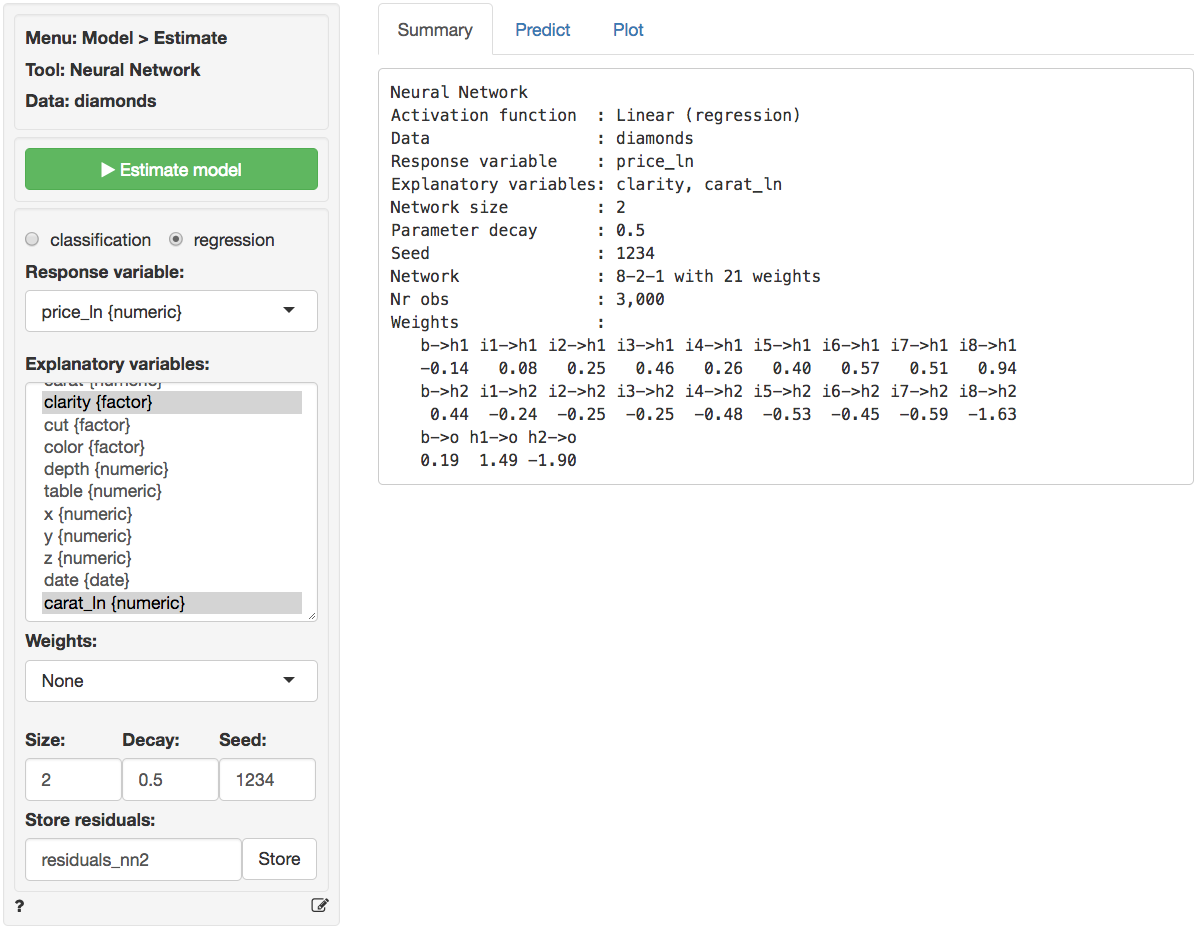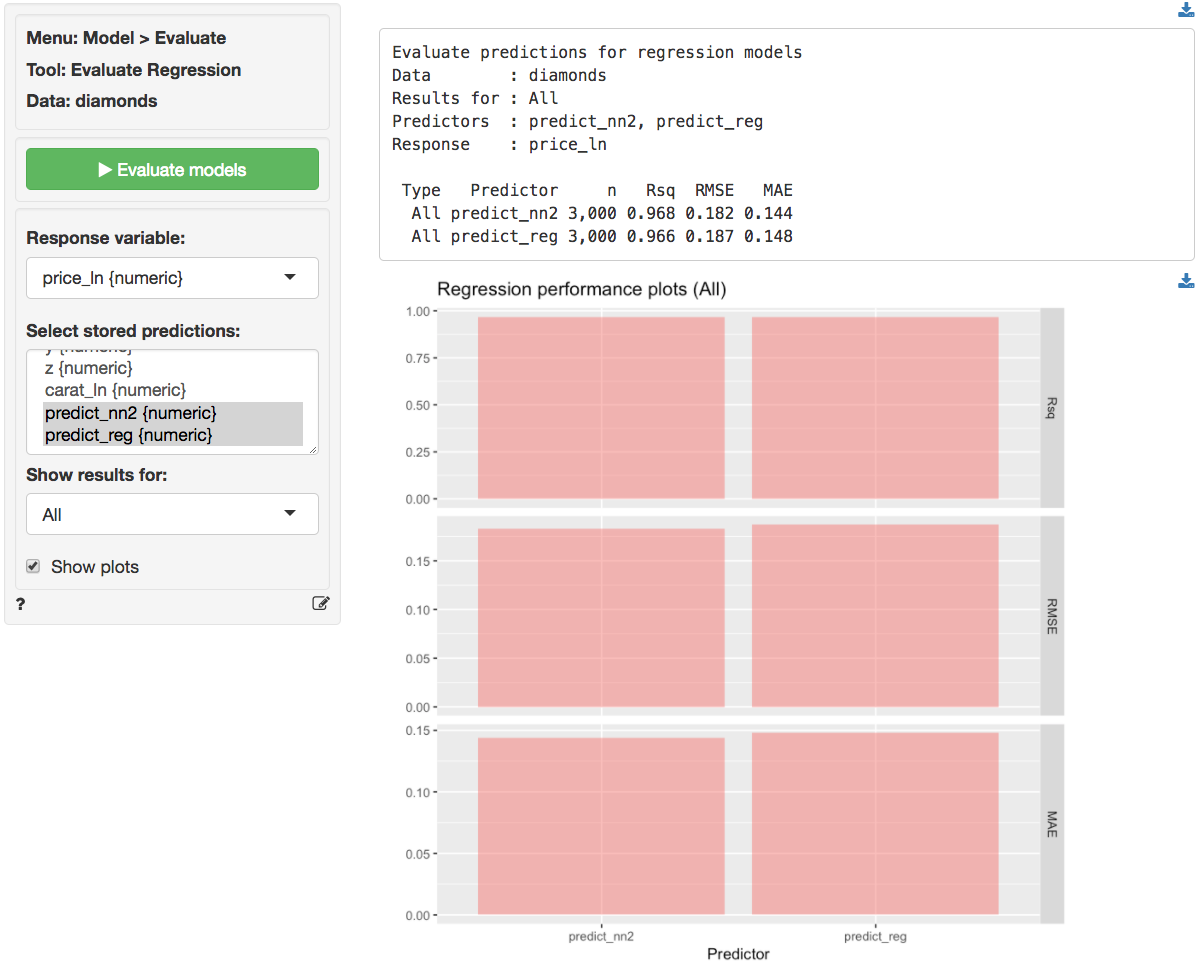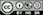Evaluate regression model performance

#### Response variable

The numeric outcome, or response, variable of interest.

#### Predictor

Select one or more variables that can be used to predict the value of the response variable. This could be a variable or predicted values from a model (e.g., from a regression estimated using Model > Linear regression (OLS) or a Neural Network estimated using Model > Neural Network).

#### Show results for

If a filter is active (e.g., set in the Data > View tab) generate results for All data, Training data, Test data, or Both training and test data. If no filter is active calculations are applied to all data.

## Example

Predictions were derived from a linear regression and an neural network with two nodes in the hidden layer on the diamonds data. The variables price and carat were log-transformed prior to estimation.The data is available through the Data > Manage tab (i.e., choose Examples from the Load data of type drop-down and press Load). The predictions shown below were generated in the Predict tab.The test statistics show a small, but consistent, advantage for the NN.### Report > Rmd

Add code to Report > Rmd to (re)create the analysis by clicking the icon on the bottom left of your screen or by pressing ALT-enter on your keyboard.

Rsq, RSME, and MAE are plotted by default. It is possible to customize the plotted results through Report > Rmd. To change the plot use, for example:

plot(result, vars = "Rsq")

The plot can be further customized using ggplot2 commands (see example below)). See Data > Visualize for details.

plot(result, vars = "Rsq") +
labs(caption = "Based on data from ...")

### R-functions

For an overview of related R-functions used by Radiant to evaluate regression models see Model > Evaluate regression

© Vincent Nijs (2019)